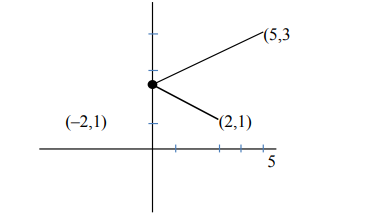# A ray of light through (2,1) is reflected at a point P on the y-axis and then passes through the point (5, 3).`
Question:

A ray of light through (2,1) is reflected at a point P on the y-axis and then passes through the point (5, 3). If this reflected ray is the directrix of an

ellipse with eccentricity $\frac{1}{3}$ and the distance of the nearer focus from this directrix is $\frac{8}{\sqrt{53}}$, then the equation of the other directrix can be:

1. $11 x+7 y+8=0$ or $11 x+7 y-15=0$

2. $11 x-7 y-8=0$ or $11 x+7 y+15=0$

3. $2 x-7 y+29=0$ or $2 x-7 y-7=0$

4. $2 x-7 y-39=0$ or $2 x-7 y-7=0$

Correct Option: , 3

Solution:Equation of reflected Ray

$y-1=\frac{2}{7}(x+2)$

$7 y-7=2 x+4$

$2 x-7 y+11=0$

Let the equation of other directrix is

$2 x-7 y+\lambda$

Distance of directrix from Focub

$\frac{a}{e}-a e=\frac{8}{\sqrt{53}}$

$3 a-\frac{a}{3}=\frac{8}{\sqrt{53}}$ or $a=\frac{3}{\sqrt{53}}$

Distance from other focus $\frac{\mathrm{a}}{\mathrm{e}}+\mathrm{ae}$

$3 a+\frac{a}{3}=\frac{10 a}{3}=\frac{10}{3} \times \frac{3}{\sqrt{53}}=\frac{10}{\sqrt{53}}$

Distance between two directrix $=\frac{2 a}{e}$

$=2 \times 3 \times \frac{3}{\sqrt{53}}=\frac{18}{\sqrt{53}}$

$\left|\frac{\lambda-11}{\sqrt{53}}\right|=\frac{18}{\sqrt{53}}$

$\lambda-11=18$ or $-18$

$\lambda=29$ or $-7$

$2 x-7 y-7=0$ or $2 x-7 y+29=0$